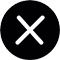News
NEWS
How to calculate DDP sea freight? Calculating formula exposure is easy to understand in secondsHow to calculate DDP sea freight? Calculating formula exposure is easy to understand in seconds

The cost calculation of DDP ocean freight is related to many factors, and the content of price calculation is also relatively complicated and difficult for people

who have never been exposed to it to understand. I will share a simplified version of the calculation formula of DDP ocean freight with you, which is easy to

understand.

Generally speaking, the calculation of DDP sea freight needs to consider the following costs:

Purchase cost: refers to the price at which the seller purchases goods from the supplier, usually based on FOB (free on board) or CIF (cif).

Domestic transportation and miscellaneous charges: refers to the expenses incurred by the seller to transport the goods from the factory or warehouse to the

port of departure, and to carry out operations such as container loading, customs declaration, and inspection.

Ocean freight: refers to the fee stipulated in the transportation contract signed between the seller and the shipping company or freight forwarding company. It

is usually calculated based on the weight or volume of the goods.

Destination port fees: refers to the fees incurred by the seller in handling import customs clearance procedures at the destination port, paying relevant import

taxes and fees, picking up the goods, and arranging services such as towing, unpacking, and local delivery.

DDP sea freight calculation formula, DDP sea freight forwarding company

The calculation formula for DDP sea freight is:

DDP = purchase cost * [(1-1/1.17*0.1) + (customs declaration and inspection + F domestic transportation and miscellaneous charges)] * (1+z*y)+ F sea

transportation + (THC + customs clearance) *z

Among them, z is the exchange rate, y is the value-added tax rate of the destination country, F is the ocean freight rate, and THC is the terminal operating fee

of the destination port.

Let’s use data as an example to give you a deeper understanding:

- For example, suppose the seller exports a batch of clothing from China to the United States. The FOB price of the clothing is US\$10,000, the freight is

US\$500, the insurance premium is US\$100, the tariff rate is 5%, the value-added tax rate is 10%, and the exchange rate is 6.5. Then DDP sea freight can be

calculated according to the following steps:How to calculate DDP sea freight? Calculating formula exposure is easy to understand in seconds

Calculate the CIF price: CIF = FOB + freight + insurance = 10,000 + 500 + 100 = 10,600 US dollars

Calculate domestic transportation and miscellaneous charges: Assume that the customs declaration and inspection fee is 2,000 yuan, and F domestic

transportation and miscellaneous charges are 0.05, then domestic transportation and miscellaneous charges = customs declaration and inspection + FOB

domestic

transportation and miscellaneous charges = 2,000 + 10,000 * 0.05 = 3,000 yuan.

Calculate the purchase cost: Purchase cost = CIF / (1-1/1.17*0.1) = 10600 / (1-0.085) = 11586.21 US dollars

Calculate the destination port fee: Assume that the THC is 200 US dollars and the customs clearance fee is 300 US dollars, then the destination port fee =

(THC + customs clearance) * z = (200 + 300) * 6.5 = 3250 yuan

Calculate DDP sea freight: DDP = purchase cost * (1+z*y) + F sea freight + destination port fee = 11586.21 * (1+6.5*0.1) + 500 + 3250 = 15736.21 US dollars

Have you learned it? The amount in the above DDP calculation formula is just a simulation and is for reference only. If you want to get an accurate quotation,

please call our Xiangcheng International Logistics service line: 4008086156